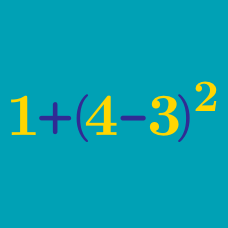Everyday Math

# Divisibility by 3

Our goal in this quiz is to solve this problem:

Justify visually that 258 is divisible by 3.

We'll start by analyzing the problem. Since the goal is a visual representation, we should try to represent 258 visually. Which of these is 258?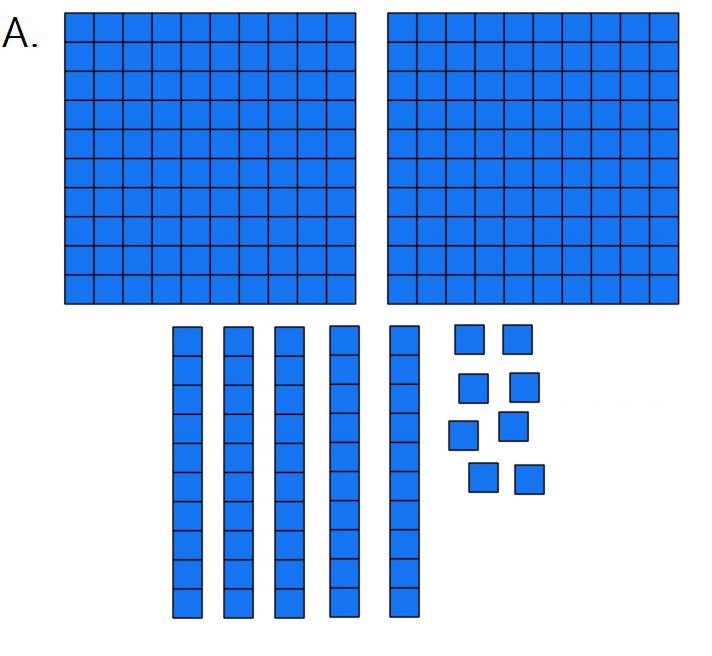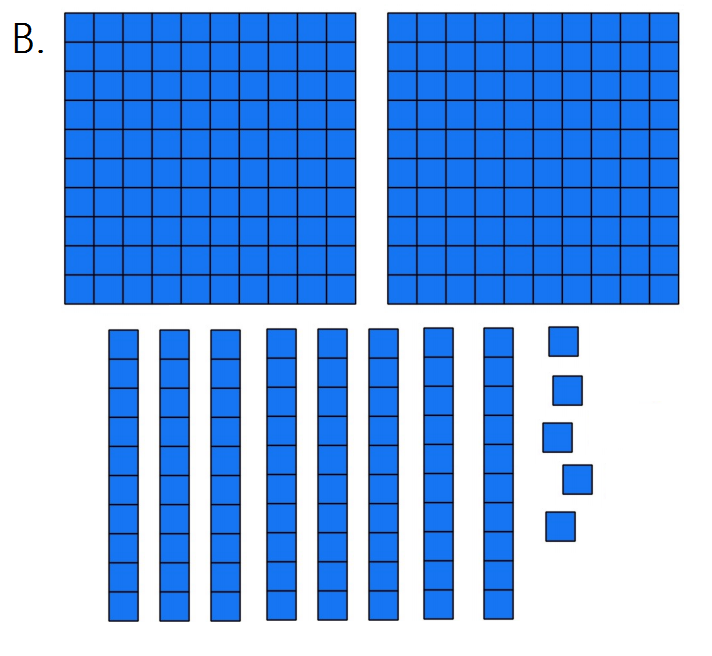Our goal is to

Justify visually that 258 is divisible by 3.

Now we'd like to explore the problem; which often is an experiment with a simpler case. Based on the diagram, why is 111 divisible by 3?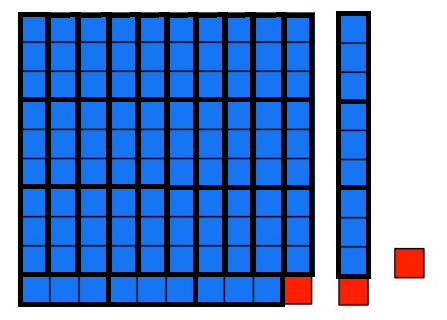You should explore and try an experiment with a number other than 111 (like 333) before answering this question (that is, make a diagram like in problem #2 and see if it justifies divisibility by 3). Usually one experiment is not enough!

Based on those experiments, plan which approach would aid justifying why 258 is divisible by 3?

Represent the number out of blocks of size 100, 10, and 1, then ...

Which of these will justify visually that 258 is divisible by 3?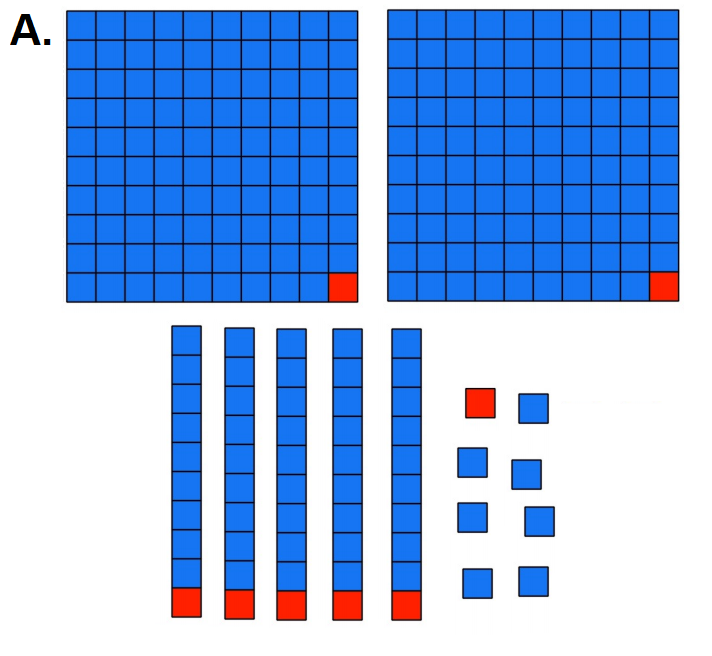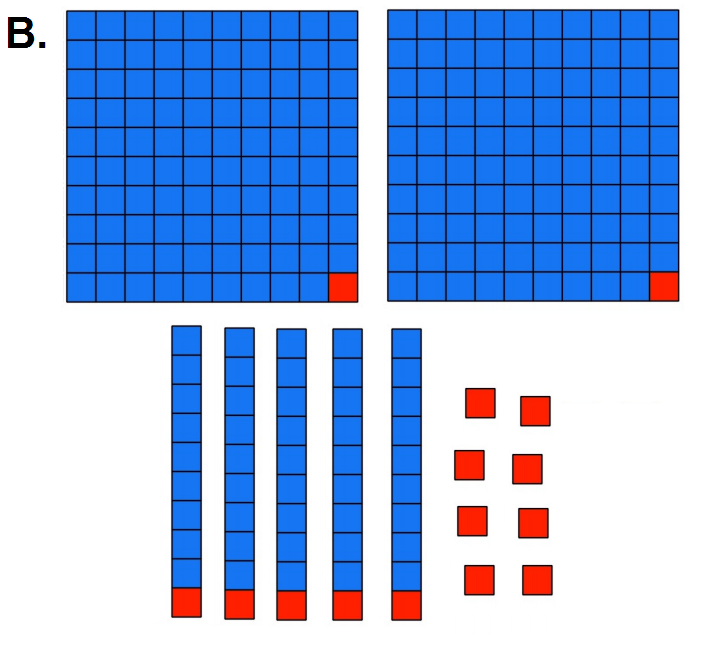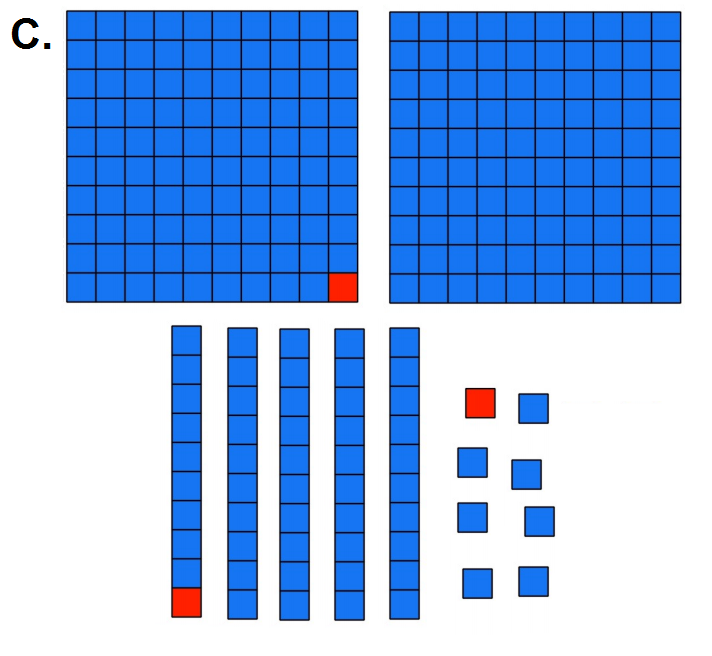After solving a problem, it's good to look back; not just to verify your answer, but to think about more general cases.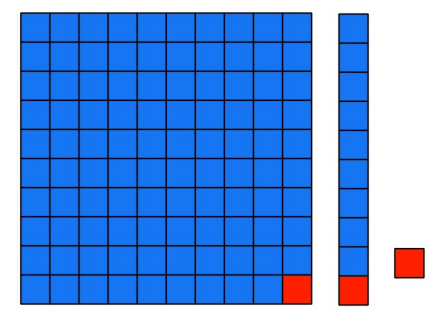Since 99 and 9 are always divisible by 3, the thing that makes a number not divisible by 3 are the red squares. If the red squares count to something divisible by 3, the whole number is divisible by 3. The red squares are also:

×